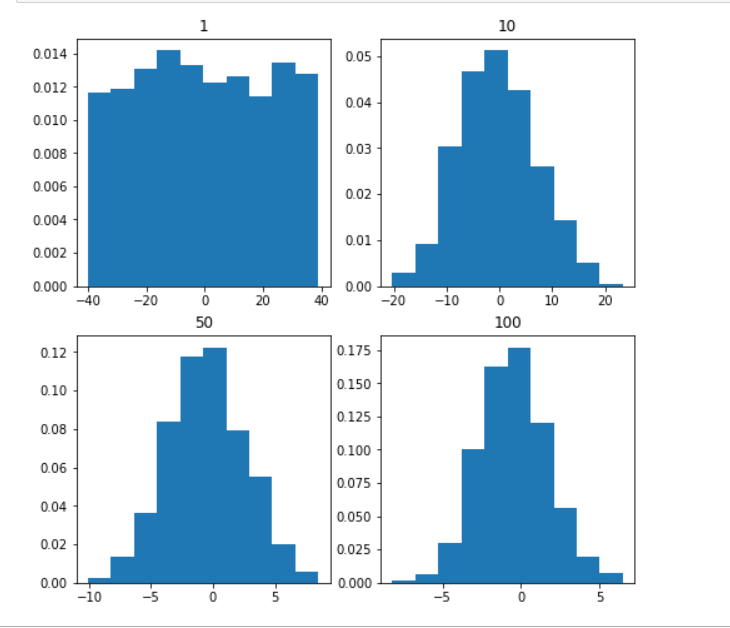top of page

# TOP 10 statistical concepts in data science

## 1)Measures of Central Tendency

#### measures of Central Tendency is a summary measure that describes a whole set of data with a single value that represents the middle or centre of its distribution. we have 3 measures of central tendency: the mode, the median and the mean

1)Mode: is the most commonly occurring value in a distribution.

2)median : is the middle value in distribution when the values are arranged in ascending or descending order.(used when there is outliers)

3)mean : is the sum values in a dataset over the the number of values in a dataset ( is sutable for unifrom dataset , affected by ouliers)

```import pandas as pd
import numpy as np
import matplotlib.pyplot as plt```

````df['ApplicantIncome'].agg([np.mean , np.median ])````df['ApplicantIncome'].hist()

plt.axvline(df['ApplicantIncome'].mean(), color='k', linestyle='dashed', linewidth=1)
plt.axvline(df['ApplicantIncome'].median(), color='r', linestyle='dashed', linewidth=1)

plt.show()````df['ApplicantIncome'].mode()`## 3)central limit theorem

### The central limit theorem (CLT) states that the distribution of sample means approximates a normal distribution as the sample size gets larger## 4)covariance and correlation

### correlation:Correlation analysis is a method of statistical evaluation used to study the strength of a relationship between two, numerically measured, continuous variables. It not only shows the kind of relation (in terms of direction) but also how strong the relationship is.

```df = pd.read_csv('loan_data_set.csv')
#the correlation between every numeric value in dataset
df.corr(method = 'kendall')``````#bringing the covariance between each numric varaibles
df.cov()```## 5)Normal distribution

### normal distribution = a probability distribution that is symmetric about the mean

```mu, sigma = 0, 0.1 # mean and standard deviation
s = np.random.normal(mu, sigma, 1000)```

```#Verify the mean and the variance:
abs(mu - np.mean(s))

``````abs(sigma - np.std(s, ddof=1))

``````#Display the histogram of the samples, along with the probability density function:
import matplotlib.pyplot as plt
count, bins, ignored = plt.hist(s, 30, density=True)
plt.plot(bins, 1/(sigma * np.sqrt(2 * np.pi)) *
np.exp( - (bins - mu)**2 / (2 * sigma**2) ),
linewidth=2, color='r')
plt.show()```## 6)population and samples

### there are 2 ways of taking samples: 1)with replacement 2)without replacement.

```sample =df.sample(n=100)## 7)Regression

regression: determine the strength of the relationship between one dependent variable (usually denoted by Y) and a series of independent variables.

Linear regression:its the relationship between a numeric depndent variable and one or more independent variables.

Logistic regression: its relationship between the binary dependent variable and one or more independent variables.

## 8)standerd devition

Standard Deviation: It is a statistic that calculates the dispersion of a data set as compared to its mean.

```sd = np.std(df['ApplicantIncome'], ddof=1)
print("Standard Deviation:", sd)```## 9)Statistical bias

Statistical bias:it calculates the differences between results and facts , Bias implies that the data selection may have been skewed by the collection criteria.

for example to investigate people's selling habits. If the sample size is not large enough, the results may not be representative of the selling habits of all the people. That is, there may be big difference between the survey results and the actual results. Therefore, understanding the source of statistical bias can help to assess whether the observed results are close to the real results.

## 10)Variance

is a measure of variability. It is calculated by taking the average of squared distance between data point and the mean. Variance tells you the degree of spread in your data set. The more spread the data, the larger the variance is in relation to the mean.

```var = np.var(df['ApplicantIncome'] ,ddof = 1)
print("Variance:", var)```